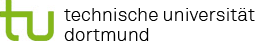You are here:

### Project R2: Regression with nonlinear modelling of metric environmental and toxicological influence factors

In project R2 flexible regression techniques will be used, which enable an automatic, data-controlled estimation of nonlinear effects of (metric) environmental and toxicological influence factors.

For the sake of simplicity, the effects of predictors are often assumed to be linear in regression (classical linear regression model). However, this is a very strong and restrictive assumption. In reality, often certain metric influence factors have more complex, not necessarily linear effects on the target variable. For example, exposure to a toxicological compound may initially have a slightly negative effect on health. However, this effect increases more than linearly (e.g. exponentially) with increasing exposure. Since the user usually does not have an exact prior knowledge of the exact form of such a nonlinear effect, it would be desirable if the form of the effect were determined automatically and data-controlled within the framework of statistical estimation.

The additive model based on smoothing splines is particularly suitable for this purpose (see, e.g., Wood, 2017 and Ruppert et al., 2003). If target distributions other than the normal distribution are used, e.g. for binary target quantities or count data, such a model is more generally called a Generalised Additive Model (GAM). In this situation, a typical procedure is to develop a corresponding metric influencing variable, which potentially has a nonlinear effect, in a number of m basic functions. A frequently used class of basic functions is the so-called Bspline basis. In order to ensure sufficient flexibility, usually a relatively large number of basic functions are selected (e.g. m=20). In order to avoid overfitting, the roughness of the spline is then penalised. A classic example are penalised B-splines (called P-splines; see Eilers and Marx, 1996). In this framework, complex nonlinear interactions can also be mapped using bivariate tensor splines.

Since it is usually difficult for the user to decide which metric influence variables should be included in the model linearly and which ones in the form of splines, questions of effect selection arise. A useful extension in this context are component-wise boosting methods for additive GAMs, which allow an automatic effect selection, so that individual covariate effects are included either linearly or nonlinearly in the model or are completely excluded (see, e.g., Hothorn et al., 2010; Groll and Tutz, 2012).

If the distribution of the target quantity depends on several parameters (e.g., expected value µ and variance σ² for the normal distribution), the model class of the GAMs can be extended so that not only the expected value as usual, but also the other distribution parameters are associated with covariates. One then obtains the extended model class of the Generalised Additive Model for Location, Scale and Shape (GAMLSS; Rigby and Stasinopoulos, 2005).

In this project, this whole range of flexible regression techniques for modelling environmental and toxicological factors influencing health will be exploited and, if necessary, meaningfully extended.

#### References

• Eilers PHC, Marx BD (1996). Flexible smoothing with B-splines and penalties. Statistical Science 11(2), 89-121, doi: 10.1214/ss/1038425655. Groll A, Tutz G (2012). Regularization for generalized additive mixed models by likelihoodbased boosting. Methods of Information in Medicine 51(2), 168-177, doi: 10.3414/ME11-020021.
• Groll A, Tutz G (2012). Regularization for generalized additive mixed models by likelihoodbased boosting. Methods of Information in Medicine 51(2), 168-177, doi: 10.3414/ME11-020021.
• Hothorn T, Bühlmann P, Kneib T, Schmid M, Hofner B (2010). Model-based Boosting 2.0. J Machine Learning Research 11, 2109-13.
• Rigby RA, Stasinopoulos DM (2005). Generalized additive models for location, scale and shape. J Royal Statistical Society: Series C (Applied Statistics) 54(3), 507-54, doi: 10.1111/j.1467-9876.2005.00510.x.
• Ruppert D, Wand MP, Carroll RJ (2003). Semiparametric regression (No. 12). Cambridge University Press.
• Wood SN (2017). Generalized additive models: an introduction with R. Chapman and Hall/CRC.